## Hensel's Lemma

An important result in Valuation Theory which gives information on finding roots of Polynomials. Hensel's lemma is formally stated as follow. Letbe a complete non-Archimedean valuated field, and letbe the corresponding Valuation Ring. Letbe a Polynomial whose Coefficients are inand supposesatisfies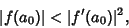(1)

where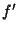is the (formal) Derivative of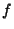. Then there exists a unique elementsuch thatand(2)

Less formally, ifis a Polynomial with Integer'' Coefficients andis small'' compared to, then the equationhas a solution near''. In addition, there are no other solutions near, although there may be other solutions. The proof of the Lemma is based around the Newton-Raphson method and relies on the non-Archimedean nature of the valuation.

Consider the following example in which Hensel's lemma is used to determine that the equationis solvable in the 5-adic numbers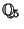(and so we can embed the Gaussian Integers insidein a nice way). Letbe the 5-adic numbers, let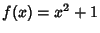, and let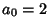. Then we haveand, so(3)

and the condition is satisfied. Hensel's lemma then tells us that there is a 5-adic numbersuch thatand(4)

Similarly, there is a 5-adic numbersuch thatand(5)

Therefore, we have found both the square roots ofin. It is possible to find the roots of any Polynomial using this technique.